## Rutherford used a 5.5 MeV alpha particle. Calculate the de Broglie wavelength of these alpha particles. How does this compare to the size of

Question

Rutherford used a 5.5 MeV alpha particle. Calculate the de Broglie wavelength of these alpha particles. How does this compare to the size of the nucleus? What if he had only been able to get alpha particles with an energy of 1 keV { would he have been able to see this e ect?

in progress 0
2 months 2021-08-04T22:29:29+00:00 1 Answers 1 views 0

Wavelength of alpha particle is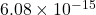m

The de broglie wavelength of 1keV is 451 times larger than size of nucleus.

Explanation:

Given:

Energy of alpha particle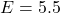MeV

De broglie wavelength corresponding the energy of alpha particle is given by,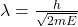Where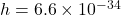Js,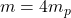,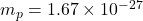kg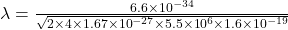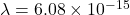m

Hence, wavelength of alpha particle ism

Size of nucleus is ≅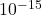m

Now wavelength of 1 keV is given by,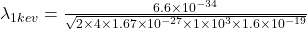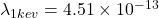m

Here,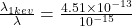≅ 451

Therefore, the de broglie wavelength of 1keV is 451 times larger than size of nucleus.